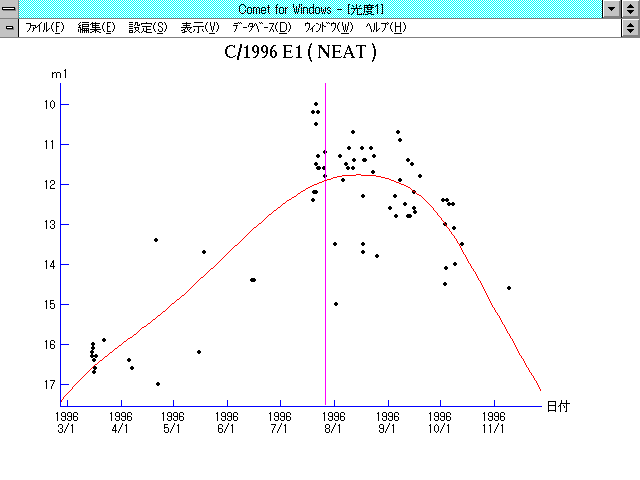# \$B%K!<%HWB@1(B

C/1996 E1 ( NEAT )###\$B%W%m%U%#!<%k(B

 \$BH/8+F|(B 1996\$BG/(B3\$B7n(B15\$BF|(B \$BH/8+8wEY(B 16.2\$BEy(B \$BH/8+ Near-Earth-Asteroid Tracking Team (Jet Propulsion Laboratory)

###\$B50F;MWAG(B

```   The following improved orbital elements, by Kenji Muraoka, are
from 230 observations 1996 Mar. 15 to Oct. 12, including 9 Planets,
Moon and 5 minor planets perturbations and non-gravitational effect
of style II.   The mean residual is +/- 0.69 arc seconds.

Epoch  =  1996 July 16.0  TT       JDT = 2450280.5
T  =  1996 July 27.34480       +/- 0.00027 (m.e.) TT
Peri. =   81.10741                +/- 0.00041
Node  =  149.84256                +/- 0.00004   (2000.0)
Incl. =  114.47558                +/- 0.00003
q  =    1.3588906              +/- 0.0000079 AU
e  =    1.0006986              +/- 0.0000081
1/a  =   -0.0005141              +/- 0.0000060 1/AU
A1  =   +7.01                   +/- 0.39
A2  =   +0.5787                 +/- 0.1266
```

###\$B@1?^(B1996\$BG/(B 1\$B7n(B 8\$BF|!A(B1996\$BG/(B 9\$B7n(B14\$BF|(B1996\$BG/(B 8\$B7n(B15\$BF|!A(B1996\$BG/(B12\$B7n(B23\$BF|(B

###\$B8wEYJQ2=(B

```        m1 = 7.4 + 5 log\$B&\$(B + 23.0 log r
```##### \$B50F;MWAG\$OB<2,7r<#;a\$N7W;;\$K\$h\$k\$b\$N\$G\$9!#(B \$B@1?^\$O(B StellaNavigator Ver.2.0 for Windows (\$B%"%9%H%m%"!<%D(B \$BJTCx(B / \$B%"%9%-!<=PHG6I4)(B) \$B\$G:n@.\$7\$?\$b\$N\$G\$9!#(B \$B8wEY%0%i%U\$O(BComet for Windows\$B\$G:n@.\$7\$?\$b\$N\$G\$9!#(B